Courses

# Test:- Complex Number - 1

## 20 Questions MCQ Test Topic-wise Tests & Solved Examples for IIT JAM Mathematics | Test:- Complex Number - 1

Description
This mock test of Test:- Complex Number - 1 for Mathematics helps you for every Mathematics entrance exam. This contains 20 Multiple Choice Questions for Mathematics Test:- Complex Number - 1 (mcq) to study with solutions a complete question bank. The solved questions answers in this Test:- Complex Number - 1 quiz give you a good mix of easy questions and tough questions. Mathematics students definitely take this Test:- Complex Number - 1 exercise for a better result in the exam. You can find other Test:- Complex Number - 1 extra questions, long questions & short questions for Mathematics on EduRev as well by searching above.
QUESTION: 1

###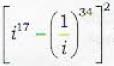is equal to

Solution: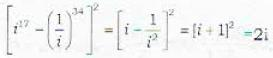QUESTION: 2

###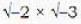is equal to

Solution: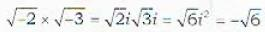QUESTION: 3

###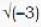x (-4) is equal to

Solution: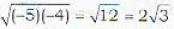QUESTION: 4

If z = 3 - 2i ; then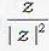equals

Solution: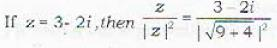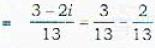QUESTION: 5

If x + iy =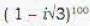, then (x, y) =

Solution: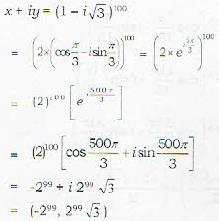QUESTION: 6

The smallest positive integer n for which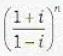is real

Solution: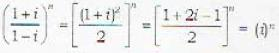so, smallest positive integer is 2 for which in will be an integer as i = -1

QUESTION: 7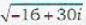is equal to

Solution:

Solution :- z = x + iy = (-16 + 30i)1/2

Squaring it we get

x2 - y2 + 2xyi = -16 + 30 i

x2 - y2 = -16……………………………….(1)

2xy = 30   =>y = 15/x

Put the value of y in eq(1)

x2- 225/x2 = -16

x4 + 16x2 -225 = 0

x4 + 25x2 - 9x2 - 225 = 0

(x2 + 25) (x2 - 9) = 0

x = +-3 and y = +-5

x + iy = +-(3 + 5i)

QUESTION: 8

Let z be a purely imaginary number such that Im(z) > 0. Then arg(z) is equal to

Solution:

Let z be the purely imaginary number other than 0 so for z = x + iy, y ≠ 0 but x = 0 so, arg will be tan-1 y/ 0 =  tan-1 ∞ = π/2

QUESTION: 9

Let z be a purely imaginary- number such that Im(z) < 0. Then arg(z) is equal to

Solution:

Let z be the purely imaginary number with (lm)z < 0, so arg(z) is, θ = - π/2

QUESTION: 10

If z be purely real number such that Re(z) < 0, then arg (z) is equal to

Solution:

Let z be the purely real number and Re(z) < 0 then arg(z) = π

QUESTION: 11

Let z be any non - zero complex number. Then arg(z) -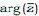is equal to

Solution:

arg(x) +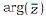= 0

QUESTION: 12

The complex number (1 + 2i)/(1 - 2i) lies in the

Solution: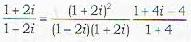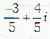i.e. in the 2nd quadrant

QUESTION: 13

i2 + i4 + i6 + .............. up to (2n + 1) terms

Solution:

i2 + i4 + i6 + ... up to (2n + 1) terms = -1
Note: For i2 + i4 + i6 + ...up to n terms
If n is even then sum = 0
If n is odd then sum = -1
hence i2 + i4 + i6 + ... (2n + 1) term is = -1

QUESTION: 14

Real part of (1 - cosθ + 2i cosθ)-1 is

Solution:

Real part of (1 - cosθ + 2: cos θ)-1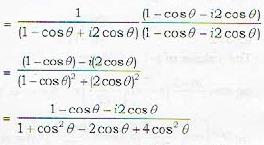So, real part is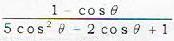QUESTION: 15

The complex number sin x + i cos 2x and cos x - i sin 2x are conjugate to each other for

Solution:

sin x + i cos 2x and cos x - i sin 2x, and their conjugates
sin x - i cos 2x and cos x + i sin 2x will be equal if
cos x: = sin x: ...(i)
=>tan.x:=1
cos 2x sin 2x: ...(ii)
=> tan 2.x = 1
tan x = tan 45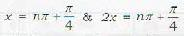which cannot be satisfied simultaneously
=> no value of x exist.

QUESTION: 16

If xand ty are non - zero real numbers such that x2 + y2 = 2 then inverse of (x + iy) is equal to -

Solution:

x2 + y2 = 2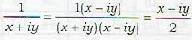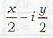QUESTION: 17

For a complex number z,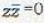, if and only if -

Solution:

Complex number z,if and only if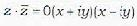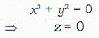QUESTION: 18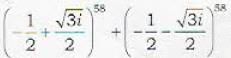is equal to

Solution: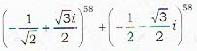= (w)58 + (w2)58 = w + w2 = 1

QUESTION: 19

The equation | z + 3 | = 6 in the complex plane represent

Solution:

| z - 3 | = 6 in the argand plane is
| z - (-3)| = 6 which is the circle where r = 6. and centre (-3,0)

QUESTION: 20

If a ≠ b, then the equation | z - a | + z + b | = 6 in the complex plane represents

Solution: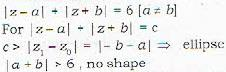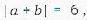,
extended portion of line segment.